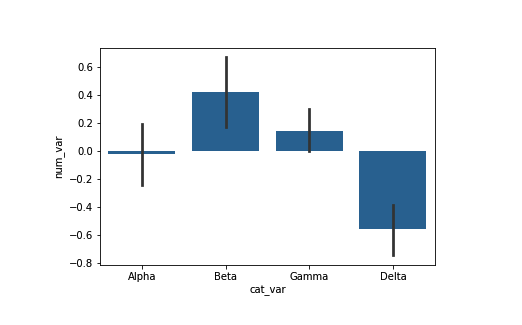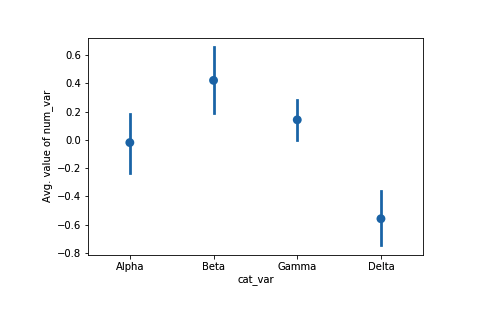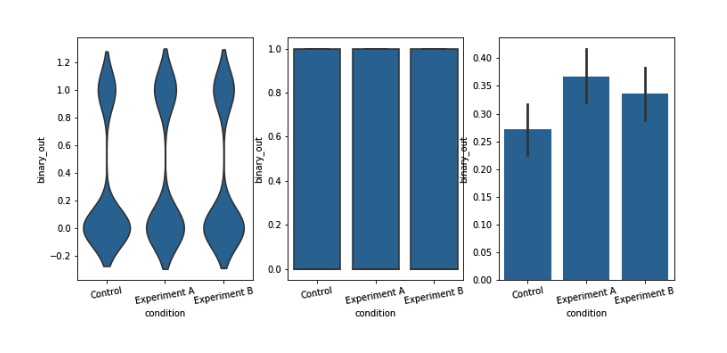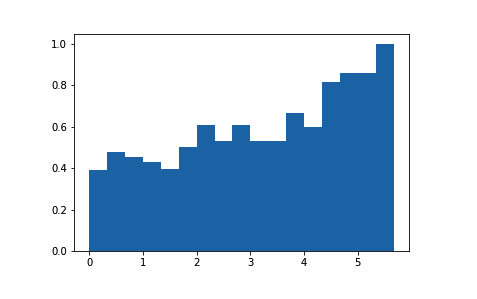# Python：matplotlib 和 Seaborn 之调整单变量图形 (三十六)

## 调整后的条形图

base_color = sb.color_palette()
sb.barplot(data = df, x = 'cat_var', y = 'num_var', color = base_color)sb.pointplot(data = df, x = 'cat_var', y = 'num_var', linestyles = "")
plt.ylabel('Avg. value of num_var')plt.figure(figsize = [12, 5])
base_color = sb.color_palette()

# left plot: violin plot
plt.subplot(1, 3, 1)
sb.violinplot(data = df, x = 'condition', y = 'binary_out', inner = None,
color = base_color)
plt.xticks(rotation = 10) # include label rotation due to small subplot size

# center plot: box plot
plt.subplot(1, 3, 2)
sb.boxplot(data = df, x = 'condition', y = 'binary_out', color = base_color)
plt.xticks(rotation = 10)

# right plot: adapted bar chart
plt.subplot(1, 3, 3)
sb.barplot(data = df, x = 'condition', y = 'binary_out', color = base_color)
plt.xticks(rotation = 10)## 调整后的直方图

bin_edges = np.arange(0, df['num_var'].max()+1/3, 1/3)

# count number of points in each bin
bin_idxs = pd.cut(df['num_var'], bin_edges, right = False, include_lowest = True,
labels = False).astype(int)
pts_per_bin = df.groupby(bin_idxs).size()

num_var_wts = df['binary_out'] / pts_per_bin[bin_idxs].values

# plot the data using the calculated weights
plt.hist(data = df, x = 'num_var', bins = bin_edges, weights = num_var_wts)